# Flow of code execution

R language constructs for flow control

Author

Pedro J. Aphalo

Published

2023-11-18

Modified

2023-11-18

Abstract

Interactive examples and flow chart diagrams for R language constructs for conditional evaluation and for repeated evaluation of code statements.

# Introduction

In a simple script the code statements are run (= executed, = evaluated) one after another as they appear in the file, from top to bottom. A simple script then always runs the same statements carrying out each time the script is run the same computations.

This restriction is removed by including control constructs that based on a test condition decide which code statement to run next. Using this constructs we can make the computations done depend on data and their properties. This is when programming starts being most useful or “powerful”, because the same code in scripts (or packages) becomes useful with many different datasets.

We can group these constructs used to control the flow of code execution from statement to statement in two groups: those that work as ON/OFF switches and those that implement the repeated execution of a statement, or iteration.

To make this work usefully, we need one more construct: a construct to assemble a compound code statement from multiple simple code statements. This makes it possible for the control constructs mentioned above to control groups of statements or “chunks” of code in addition to simple statements.

Below, we start with the sequential execution of statements in their “natural” ordering and grouping into compound statements. Next, we look and the ON/OFF or YES/NO constrtucts (if, if ... else and ifelse). Last we look at the iteration or “repeated execution” control constructs (for (), while() and repeat).

Playgrounds or interactive R examples

Within this page you will run R examples in the web browser. You can edit these code examples and run them again as many times as you like, by clicking on the Run Code button above the ‘WebR’ text panels.

# Unconditional execution

## Sequence

Code
a <- 123
b <- a * 2
print(b)
 246
Playground

## Compound statement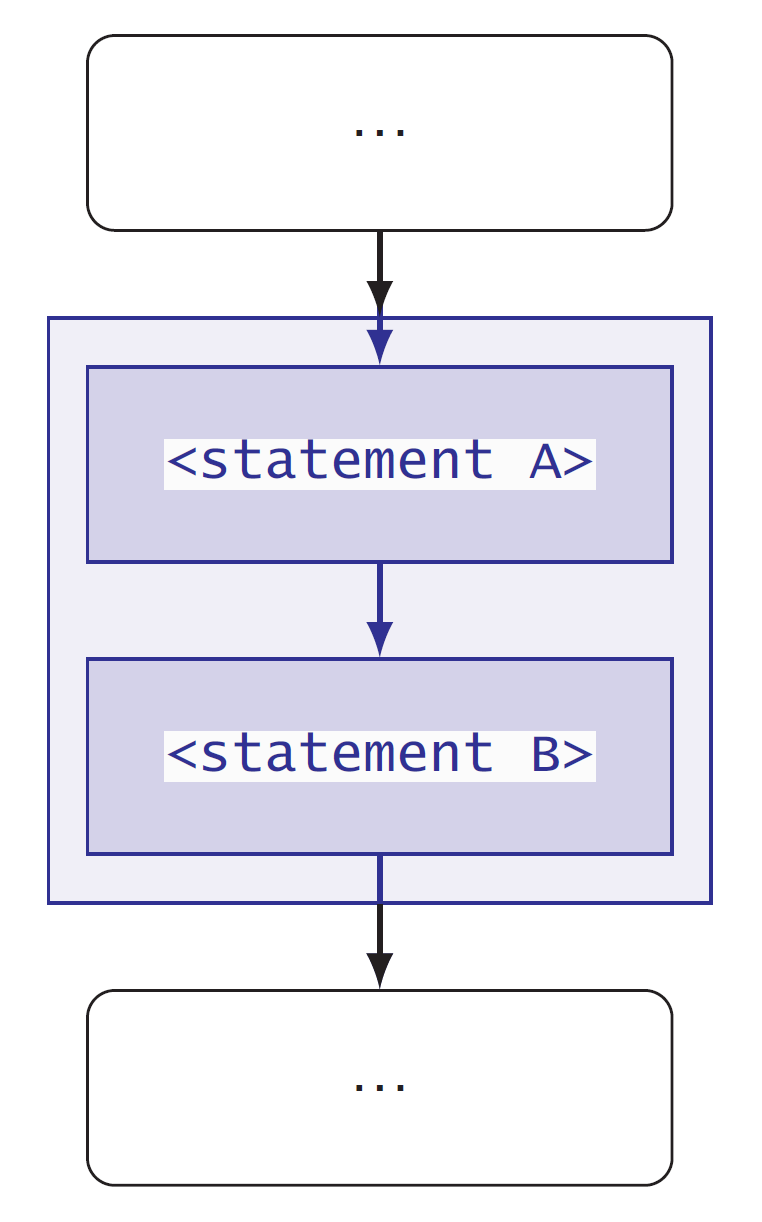A compound statement containing two statements and their sequence of evaluation..

A group code statements enclosed in { } conform a compoud statement. By itself this construct does not modify the sequence in which statements are executed or evaluated.

Code
a <- 123
{
b <- a * 2
print(b)
}
 246
Playground

# Conditional execution

Decisions about which statement to run next are based on the result of a test returning a logical value, TRUEorFALSE.

## if () statement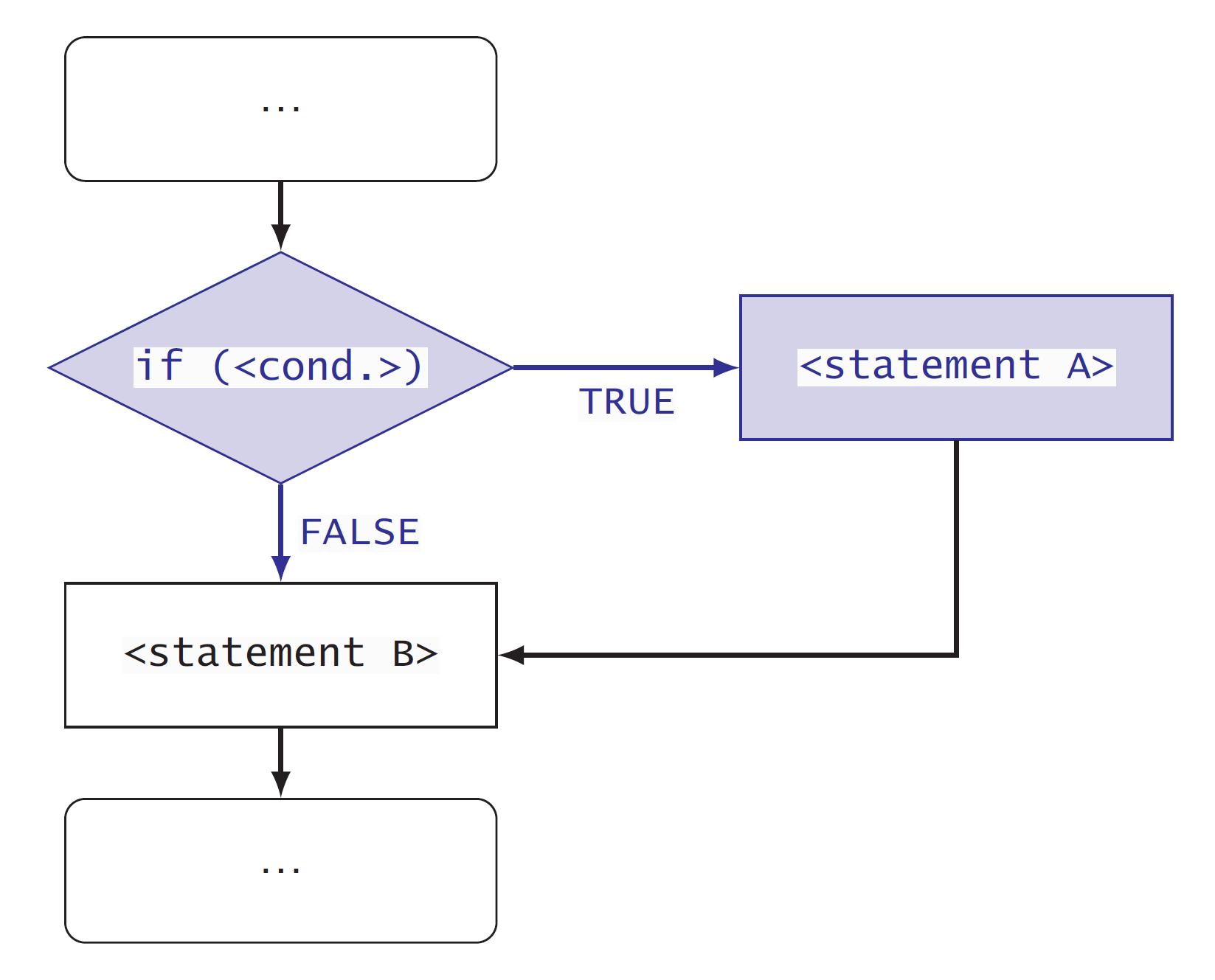Flow diagram of an if () control construct showing the alternative paths of evalaution.

In an if statement, the decision is based on the value of a logical vector of length one.

Code
a <- 123
if (a > 0) {
print("'a' is positive")
}
 "'a' is positive"
Code
print(a)
 123
Playground

## if ()... else statement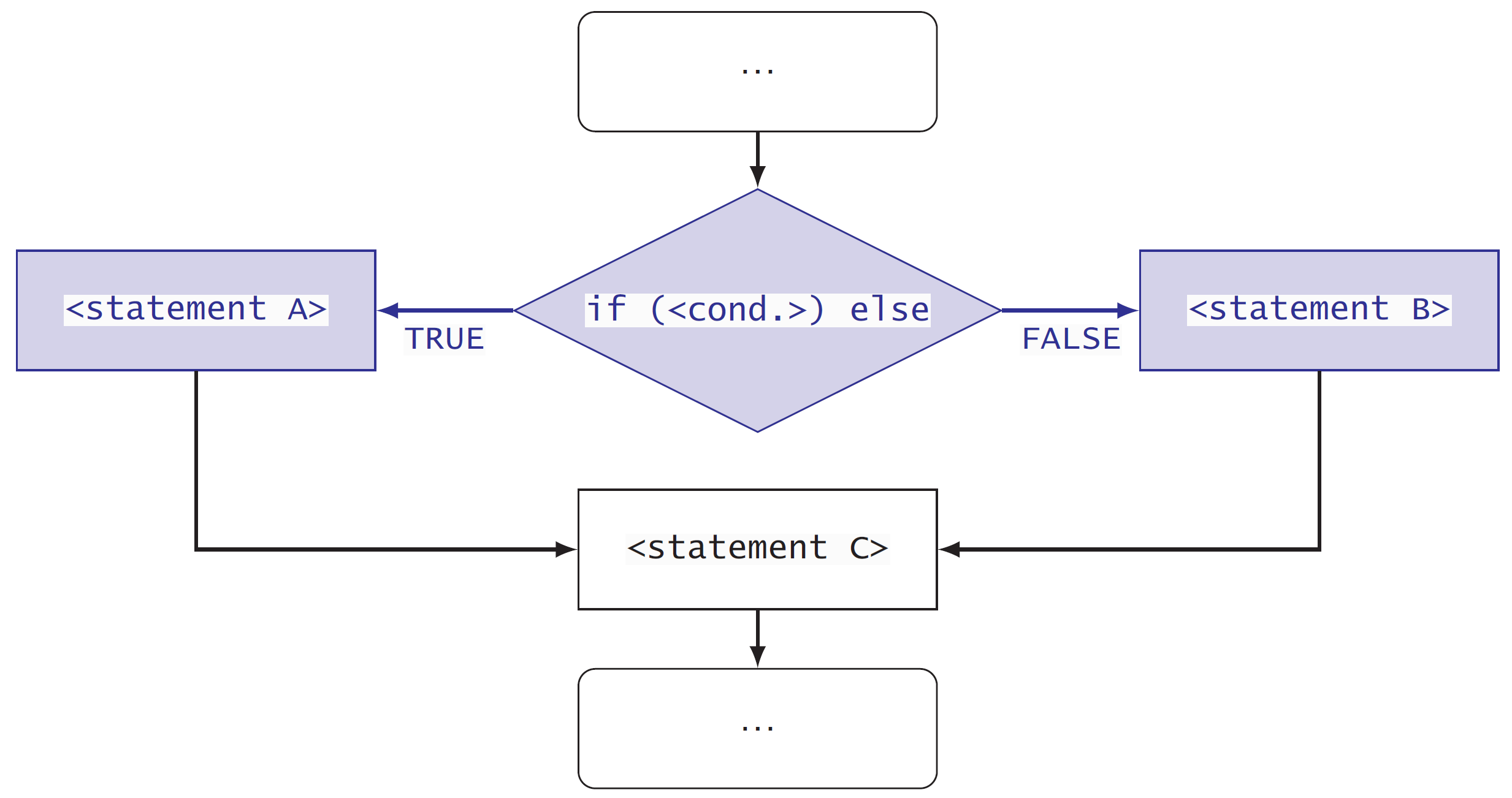Flow diagram of an if () control construct showing the alternative paths of evaluation.
Code
a <- 123
if (a >= 0) {
print("'a' is positive")
} else {
print("'a' is negative")
}
 "'a' is positive"
Code
print(a)
 123
Playground

For dealing with vectors longer that one we can use all() and any(). Of course, if they describe the decision we need to process our data.

An advanced/more sophisticated alternative is to count the number of members in the vector that are positive and negative.

## switch statement

In a switch statement the decision selects the evaluation of one among multiple alternative staements. The “selector” can be an integer or a character vector of length equal to one.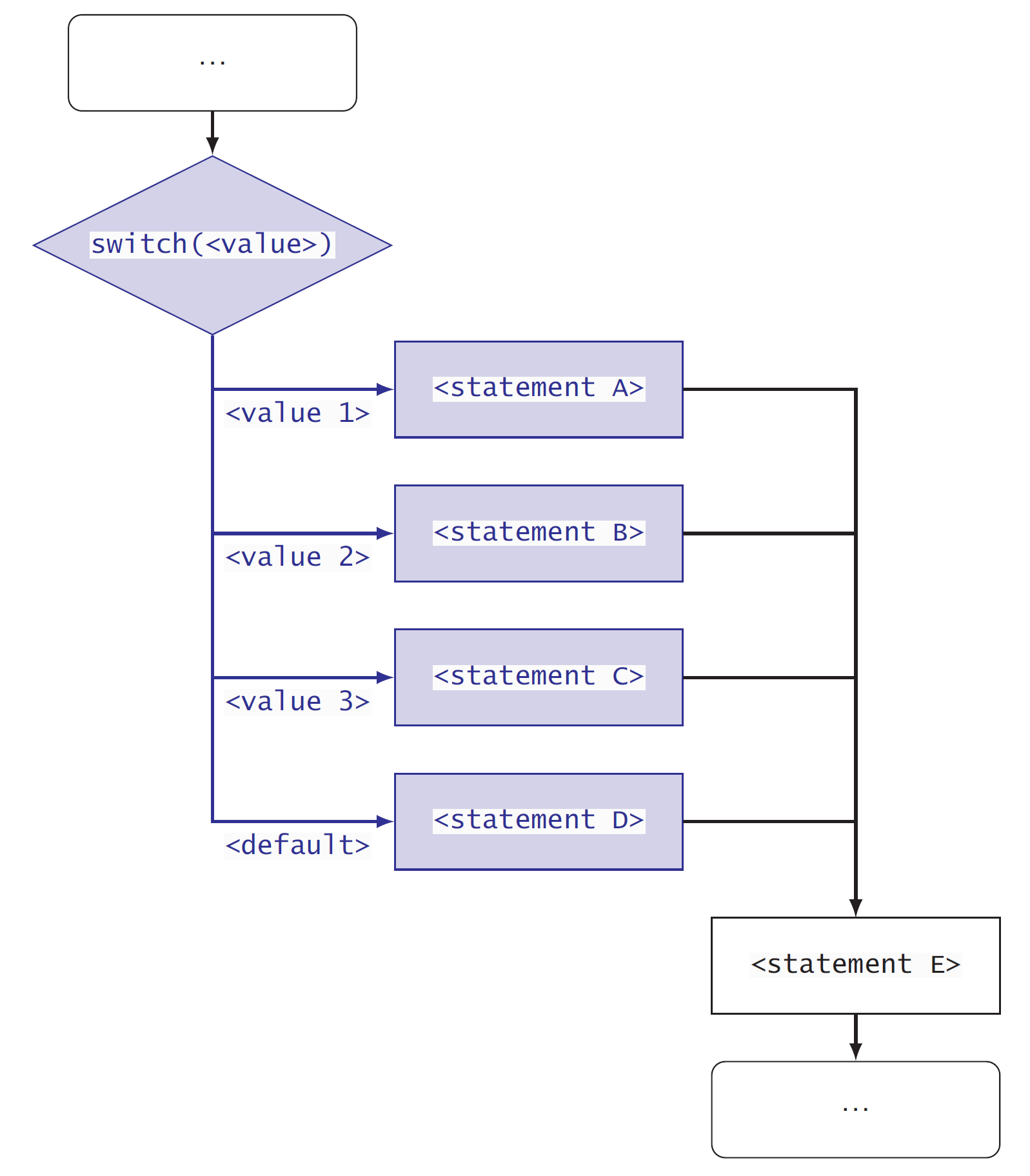Flow diagram showing multiple alternative code statements with evaluation controlled by a switch() statement.

Using names for the cases in the switch.

Code
my.char <- "two"
my.fraction <- switch(my.char,
one = 1,
two = 1 / 2,
four = 1 / 4,
0
)
print(my.fraction)
 0.5
Playground

Using numbers to select cases by position.

Playground

## ifelse(...)vectorised statement

Function ifelse() works very differently than if () ... else. The decision can, and in most cases, it is based on a long logical vector. The returned value has the same length as the logical vector.

Code
a <- c(1, 2, -1, 3) # a vector of length 3
ifelse(a >= 0, "positive", "negative")
 "positive" "positive" "negative" "positive"
Code
print(a)
  1  2 -1  3
Playground

# Iteration or repeated execution

## for () statementFlow diagram of a for () loop, showing the compound statement that is evaluated repeatedly.

In a for statement, the controlled statement is run with one member of a list or vector at a time, normally walking from “head” to “tail” of the list or vector. The value of each member is accessed through a “place holder” variable. The name of the arbitrary variable can be any valid R name.

Code
a <- c(1, 2, -1, 3)
for (a_member in a) {
print(a_member)
}
 1
 2
 -1
 3
Code
print(a)
  1  2 -1  3
Playground

Using extraction operator [ ] and indexing.

We can end early.

## while() statement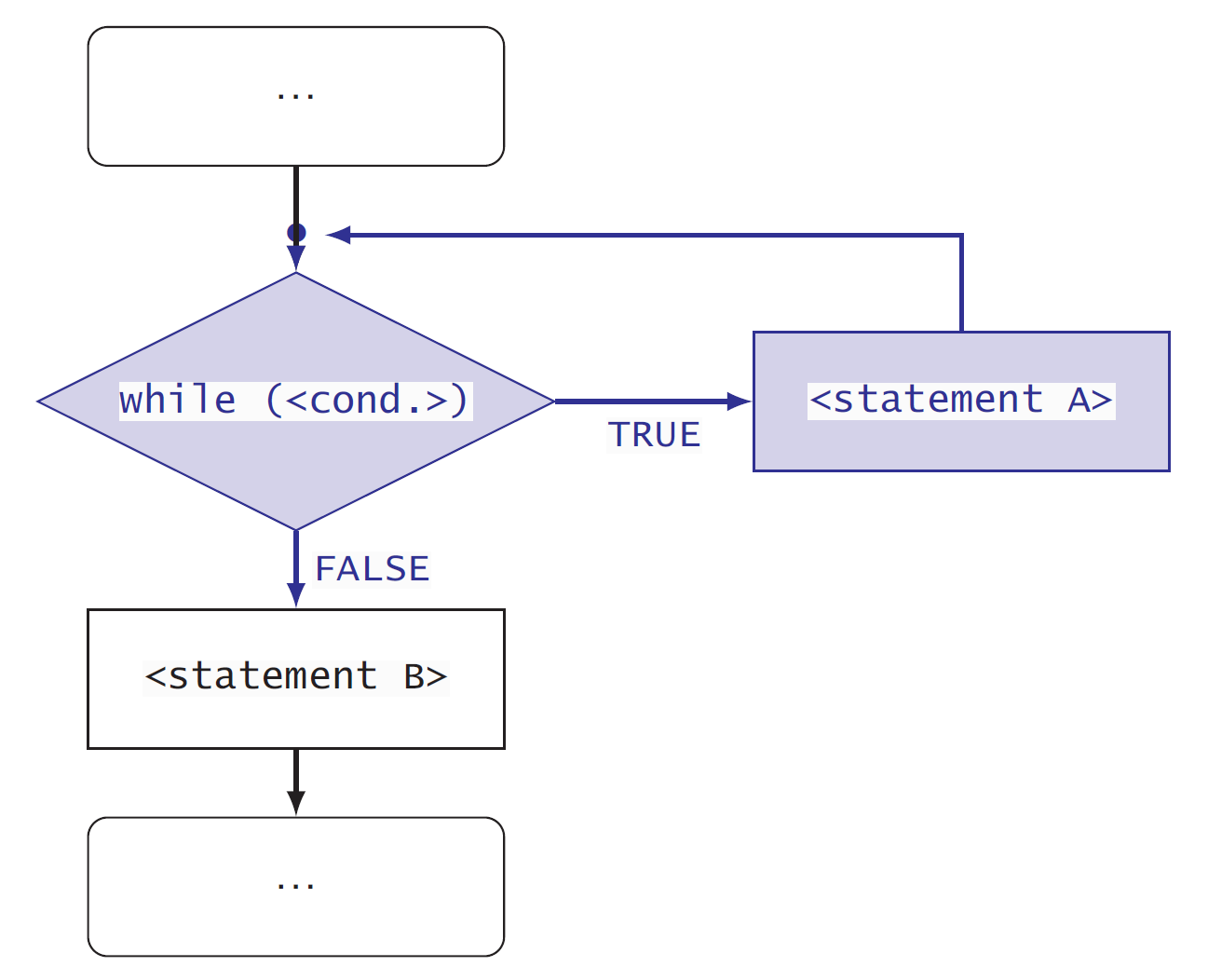Flow diagram of an while() control construct showing the closed loop containing a statement repeatedly evaluated.
Code
a <- c(1, 2, -1, 3)
i <- 0
sum_of_a <- 0
while(i < length(a)) {
i <- i + 1
sum_of_a <- sum_of_a + a[i]
print(sum_of_a)
}
 1
 3
 2
 5
Code
print(i)
 4
Playground

## repeat statement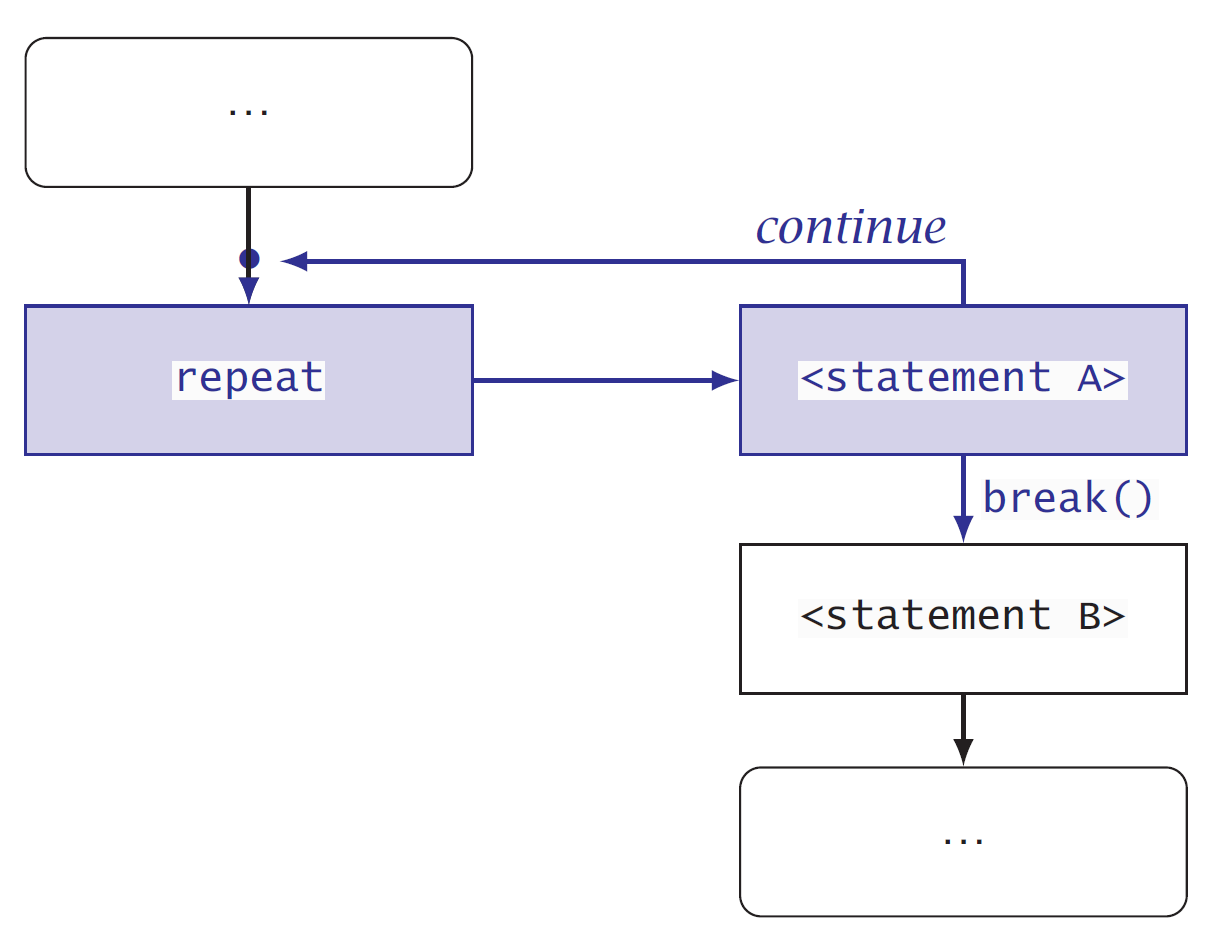Flow diagram of an repeat control construct showing one statement repeatedly evaluated in a loop-shaped path
Code
a <- c(1, 2, -1, 3)
i <- 0
sum_of_a <- 0
while(i < length(a)) {
i <- i + 1
sum_of_a <- sum_of_a + a[i]
print(sum_of_a)
}
 1
 3
 2
 5
Code
print(i)
 4`
Playground

# Take home message

Now you know all the important constructs that make it possible to control the flow of evaluation of statements in a script or program. With small variations in their syntax, these same constructs are available in many different computer programming languages.

That all these constructs are available in R, makes of R a proper programming language. It also means that learning to program in additional computer languages is much easier than learning the first one.